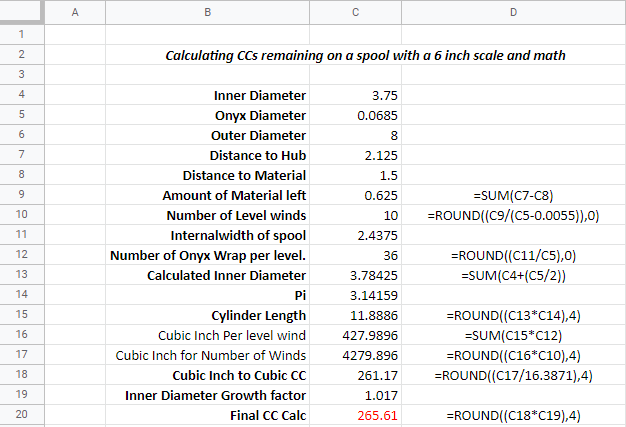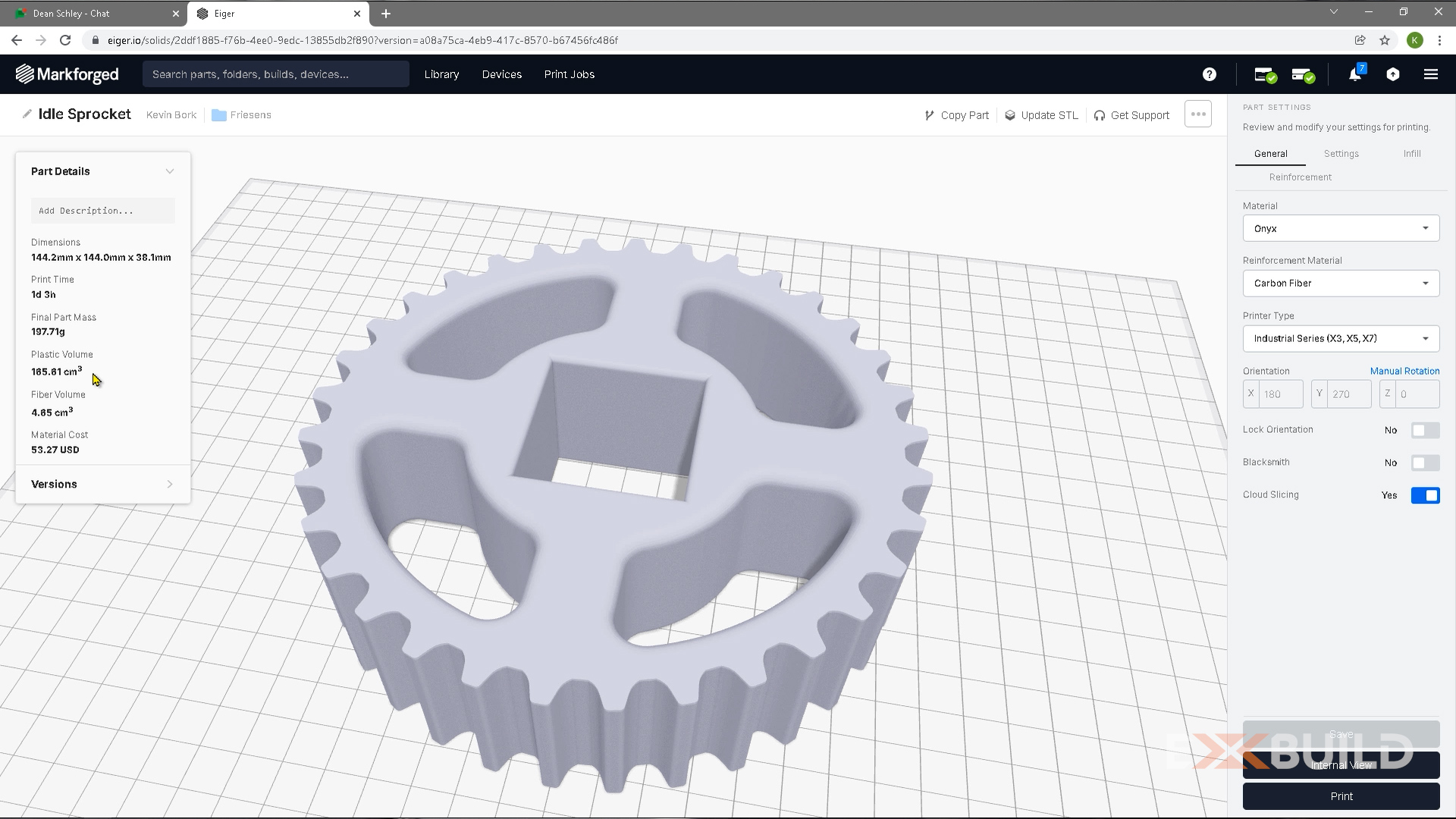# EXBuild Blog

### Posts by Topic

One question I get from many customers is "how do I calculate how many CC’s of material are left on a partial spool of material?" In our previous blog we came up with a way to calculate just that with a ruler and a scale, but what if we don't have a scale? This is a good option, a little less accurate but in the ballpark.

Step 1: Measure from the outer edge of the spool to the top of the material. Let’s say we measured 1.5.
Take 2.125-1.5 =.625

Step 2: Now we need to divide .625 /.063 = 9.9206. This would say there are 10 level passes of material on the spool.

Step 3: Side to side we take 2.4375 / .068 = 35.8455 This would say 36 wraps per diameter

### The calculation for CCs is thisStep 5:
Inner diameter of the first wrap of material times pi x 36 x 10 / cc conversion x 1.017

Step 6: 3.78425 * 3.14159 = 11.8886

Step 7: 11.8886 * 36 = 427.9896

Step 8: 427.9896 * 10 = 4279.986

Step 9: 4279.986 / 16.3871 = 261.17 CC’s of material

Step 10: 261.17 * 1.017 = 265.61 CC’s of material on the spool.Now review the Part Details in Eiger of the part you want to print. Print Volume is how many ccs are required smaller than 213 your good to go. There will be more on the spool than the above calculation as the diameter is exponential growth, and this calculation is linear.
We use .063 for a diameter value in the wrap calculation as the levels will build with all diameters tangent